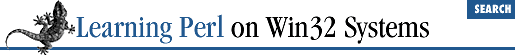home | O'Reilly's CD bookshelfs | FreeBSD | Linux | Cisco | Cisco Exam

### 7.6 The split and join Functions

Regular expressions can be used to break a string into fields. The ``` split``` function does this and the ``` join``` function glues the pieces back together.

### 7.6.1 The split Function

The ``` split``` function takes a regular expression and a string and looks for all occurrences of the regular expression within that string. The parts of the string that don't match the regular expression are returned in sequence as a list of values. For example, here's something to parse semicolon-separated fields, such as the ``` PATH``` environment variable:

```\$line = "c:\\;;c:\\windows\\;c:\\windows\\system;";
@fields = split(/;/,\$line); # split \$line, using ; as delimiter
# now @fields is ("c:\", "", "c:\windows","c:\windows\system")```

Note how the empty second field became an empty string. If you don't want this to happen, match all of the semicolons in one fell swoop:

`@fields = split(/;+/, \$line);`

This matches one or more adjacent semicolons together, so that there is no empty second field.

One common string to split is the ``` \$_``` variable, and that turns out to be the default:

```\$_ = "some string";
@words = split(/ /); # same as @words = split(/ /, \$_);```

For this split, consecutive spaces in the string to be split will cause null fields (empty strings) in the result. A better pattern would be ``` / +/``` , or ideally ``` /\s+/``` , which matches one or more whitespace characters together. In fact, this pattern is the default pattern,[ 8 ] so if you're splitting the ``` \$_``` variable on whitespace, you can use all the defaults and merely say:

 Actually, the ``` ""``` string is the default pattern, and this will cause leading whitespace to be ignored, but that's still close enough for this discussion.

`@words = split; # same as @words = split(/\s+/, \$_);`

Empty trailing fields do not normally become part of the list. This rule is not generally a concern. A solution like this:

```\$line = "c:/;c:/windows;c:/windows/system;";
(\$first, \$second, \$third, \$fourth) =
split(/;/,\$line); # split \$line, using ; as delimiter```

would simply give ``` \$fourth``` a null ( ``` undef``` ) value if the line isn't long enough, or if it contained empty values in the last field. (Extra fields are silently ignored, because list assignment works that way.)

### 7.6.2 The join Function

The ``` join``` function takes a list of values and glues them together with a glue string between each list element. The function looks like this:

`\$bigstring = join(\$glue,@list);`

For example, to rebuild the ``` PATH``` line, try something like:

`\$outline = join(";", @fields);`

Note that the glue string is not a regular expression - just an ordinary string of zero or more characters.

If you need to get glue ahead of every item instead of just between items, a simple cheat suffices:

`\$result = join("+", "", @fields);`

Here, the extra ``` ""``` is treated as an empty element, to be glued together with the first data element of ``` @fields``` . This change results in glue ahead of every element. Similarly, you can get trailing glue with an empty element at the end of the list, like so:

`\$output = join ("\n", @data, "");`